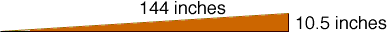Quandaries and Queries Kyle, student, middle school. What is the degree of incline of a 12 foot plank that goes from 10.5 inches on one end to zero inches on the other? Thank-you Hi Kyle, You need some trigonometry to answer this question. I first drew a diagram.From the diagram the angle of incline has sine of 10.5/144 = 0.07292 Thus the angle you want is the angle which has a sine of 0.07292. This function is called the inverse sine function and is probably accessible using the "sin-1" button on your calculator. I found the inverse sine of 0.07292 to be 4.2 degrees. Penny Go to Math Central